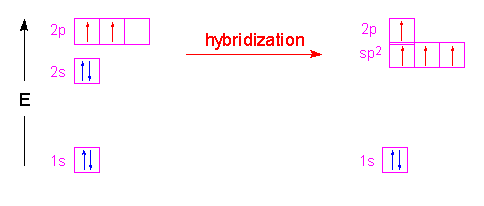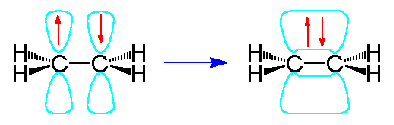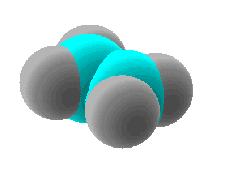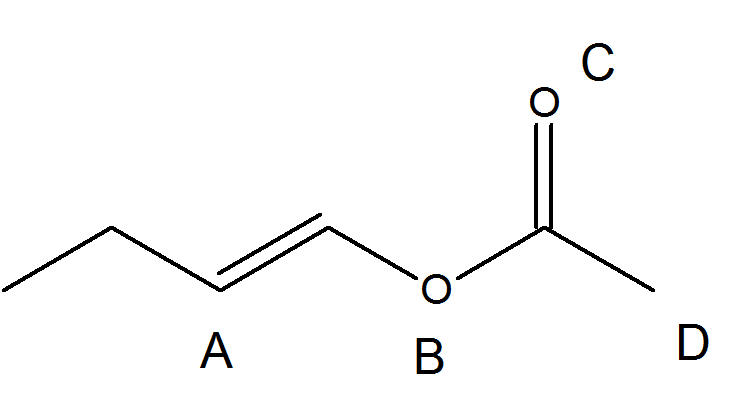## sp^2 Hybrid Orbitals and the Structure of Ethylene

### Objectives

After completing this section, you should be able to

1. account for the formation of carbon-carbon double bonds using the concept of sp2 hybridization.
2. describe a carbon-carbon double bond as consisting of one σ bond and one π bond.
3. explain the difference between a σ bond and a π bond in terms of the way in which p orbitals overlap.

### Key Terms

Make certain that you can define, and use in context, the key terms below.

• pi (π) bond
• sp2 hybrid

### Bonding in Ethene

A key component of using Valence Bond Theory correctly is being able to use the Lewis dot diagram correctly. Ethene has a double bond between the carbons and single bonds between each hydrogen and carbon: each bond is represented by a pair of dots, which represent electrons. Each carbon requires a full octet and each hydrogen requires a pair of electrons. The correct Lewis structure for ethene is shown below:

In the molecule ethene, both carbon atoms will be sp2hybridized and have one unpaired electron in a non-hybridized p orbital.These p-orbitals will undergo parallel overlap and form one $\sigma$ bond with bean-shaped probability areas above and below the plane of the six atoms. This pair of bean-shaped probability areas constitutes one $\pi$-bond and the pair of electrons in this bond can be found in either bean-shaped area.The 3-dimensional model of ethene is therefore planar with H-C-H and H-C-C bond angles of 120o…the π-bond is not shown in this picture.Valence Shell Electron Pair Repulsion (VSEPR) Theory is used to predict the bond angles and spatial positions of the carbon and hydrogen atoms of ethene and to determine the bond order of the carbon atoms (the number of bonds formed between them). Each carbon atom is of the general arrangement AX3, where A is the central atom surrounded by three other atoms (denoted by X); compounds of this form adopt trigonal planar geometry, forming 120 degree bond angles. In order for the unhybridized p orbitals to successfully overlap, the CH​2 must be coplanar: therefore, C2H4 is a planar molecule and each bond angle is about 120 degrees. The diagram below shows the bond lengths and hydrogen-carbon-carbon bond angles of ethene:

According to valence bond theory, two atoms form a covalent bond through the overlap of individual half-filled valence atomic orbitals, each containing one unpaired electron. In ethene, each hydrogen atom has one unpaired electron and each carbon is sp2 hybridized with one electron each sp​2 orbital. The fourth electron is in the p orbital that will form the pi bond. The bond order for ethene is simply the number of bonds between each atom: the carbon-carbon bond has a bond order of two, and each carbon-hydrogen bond has a bond order of one.

### Example

Consider the following molecule:At each atom, what is the hybridization and the bond angle? At atom A draw the molecular orbital.